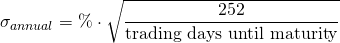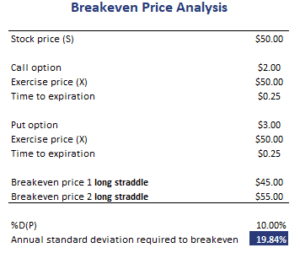# Break-even Price Analysis

Break-even Price Analysis for option strategies allows investors to determine the amount of volatility needed to earn a profit, given the break-even price of the option strategy. Thus, to apply break-even analysis, we first need to determine the break-even price of, say, a long straddle strategy. We can then use that break-even price to determine the amount of volatility needed to make a profit.

On this page, we discuss the formula that can be used to determine the amount of volatility needed to break-even. We also apply this break-even volatility methodology in the case of a long straddle strategy. A spreadsheet that implements the example is available for download at the bottom of the page.

## Break-even Price analysis formula

The formula that we can use to determine the amount of annual volatility needed to make a profit is the following.where

*** QuickLaTeX cannot compile formula:
$$\DeltaP = \frac{|{\textrm{breakeven price - current level|}}{\textrm{current price}}$$

*** Error message:
Undefined control sequence \DeltaP.
leading text: \DeltaP
File ended while scanning use of \frac .
Emergency stop.



## Break-even price analysis example

Let’s consider an example of how we can use break-even analysis for option strategies. Let’s apply the methodology to a long straddle strategy. This strategy is a very good example because it is implemented by traders when they expect volatility to be higher than implied by option prices. The following table implements the above formulas. The spreadsheet is available for download at the bottom of the page.The same method can be used to perform a break-even analysis in the case of other option strategies. For every strategy, we first need to determine the appropriate break-even price. Once we have that price, the above breakeven analysis formula can always be applied, whatever strategy we are considering.

## Summary

We discussed how we can use the break-even price of an option strategy to calculate the amount of volatility that is necessary to make the strategy profitable. The method can be used for calendar spreads, bear/bull spreads, straddles, strangles, and many other option strategies.